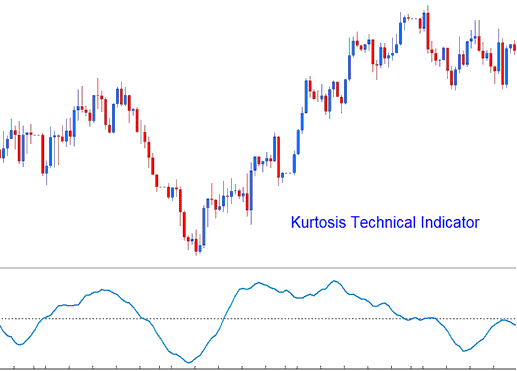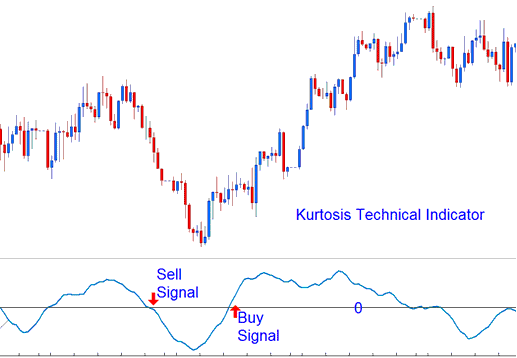# Kurtosis XAUUSD Technical Analysis and Kurtosis XAUUSD Signals

This xauusd indicator is used to help determine the sentiment of the gold market.

It is calculated in 3 parts.

• Kurtosis portion
• Fast Kurtosis
• Fast/Slow Kurtosis

The Kurtosis portion is calculated using the formula:

K = n-periods momentum - n-periods momentum of 1 bar before (current gold price bar’s momentum value minus the previous gold price bar’s momentum value)

The Fast Kurtosis portion is calculated using the formula:

FK = n-periods moving average of K

The Fast/Slow Kurtosis portion is calculated using the formula:

FSK = n-periods moving average of FK## XAUUSD Technical Analysis and Generating XAUUSD Signals

Bullish Signal - A buy xauusd signal is generated when the indicator crosses above zero.

Bearish Signal - A sell xauusd signal is generated when the indicator crosses below zero.Technical Analysis